EXACT Statement

EXACT statistic-options </ computation-options> ;

The EXACT statement requests exact tests and confidence limits for selected statistics. The statistic-options identify which statistics to compute, and the computation-options specify options for computing exact statistics. See the section Exact Statistics for details.

Note: PROC FREQ computes exact tests by using fast and efficient algorithms that are superior to direct enumeration. Exact tests are appropriate when a data set is small, sparse, skewed, or heavily tied. For some large problems, computation of exact tests might require a considerable amount of time and memory. Consider using asymptotic tests for such problems. Alternatively, when asymptotic methods might not be sufficient for such large problems, consider using Monte Carlo estimation of exact p-values. You can request Monte Carlo estimation by specifying the MC computation-option in the EXACT statement. See the section Computational Resources for more information.

Statistic Options

The statistic-options specify which exact tests and confidence limits to compute. Table 38.6 lists the available statistic-options and the exact statistics that are computed. Descriptions of the statistic-options follow the table in alphabetical order.

For one-way tables, exact p-values are available for binomial proportion tests, the chi-square goodness-of-fit test, and the likelihood ratio chi-square test. Exact (Clopper-Pearson) confidence limits are available for the binomial proportion.

For two-way tables, exact p-values are available for the following tests: Pearson chi-square test, likelihood ratio chi-square test, Mantel-Haenszel chi-square test, Fisher’s exact test, Jonckheere-Terpstra test, and Cochran-Armitage test for trend. Exact p-values are also available for tests of the following statistics: Pearson correlation coefficient, Spearman correlation coefficient, Kendall’s tau-b, Stuart’s tau-c, Somers’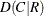, Somers’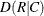, simple kappa coefficient, and weighted kappa coefficient.

Fortables, PROC FREQ provides the exact McNemar’s test, exact confidence limits for the odds ratio, and Barnard’s unconditional exact test for the risk (proportion) difference. PROC FREQ also provides exact unconditional confidence limits for the risk difference and for the relative risk (ratio of proportions). For stratifiedtables, PROC FREQ provides Zelen’s exact test for equal odds ratios, exact confidence limits for the common odds ratio, and an exact test for the common odds ratio.

Most of the statistic-option names listed in Table 38.6 are identical to the corresponding option names in the TABLES and OUTPUT statements. You can request exact computations for groups of statistics by using statistic-options that are identical to the TABLES statement options CHISQ, MEASURES, and AGREE. For example, when you specify the CHISQ statistic-option in the EXACT statement, PROC FREQ computes exact p-values for the Pearson chi-square, likelihood ratio chi-square, and Mantel-Haenszel chi-square tests for two-way tables. You can request an exact test for an individual statistic by specifying the corresponding statistic-option from the list in Table 38.6.

Using the EXACT Statement with the TABLES Statement

You must use a TABLES statement with the EXACT statement. If you use only one TABLES statement, you do not need to specify the same options in both the TABLES and EXACT statements; when you specify a statistic-option in the EXACT statement, PROC FREQ automatically invokes the corresponding TABLES statement option. However, when you use an EXACT statement with multiple TABLES statements, you must specify options in the TABLES statements to request statistics. PROC FREQ then provides exact tests or confidence limits for those statistics that you also specify in the EXACT statement.

Table 38.6: EXACT Statement Statistic Options

Statistic Option

Exact Statistics

McNemar’s test (fortables), simple kappa test,

weighted kappa test

Barnard’s test (fortables)

Binomial proportion tests for one-way tables

Chi-square goodness-of-fit test for one-way tables;

Pearson chi-square, likelihood ratio chi-square, and

Mantel-Haenszel chi-square tests for two-way tables

Confidence limits for the common odds ratio,

common odds ratio test (for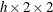tables)

Zelen’s test for equal odds ratios (fortables)

Fisher’s exact test

Jonckheere-Terpstra test

Test for the simple kappa coefficient

Test for Kendall’s tau-b

Likelihood ratio chi-square test (one-way or two-way tables)

McNemar’s test (fortables)

Tests for the Pearson correlation and Spearman correlation,

confidence limits for the odds ratio (fortables)

Mantel-Haenszel chi-square test

Confidence limits for the odds ratio (fortables)

Pearson chi-square test

Test for the Pearson correlation coefficient

Confidence limits for the relative risk (fortables)

Confidence limits for the proportion difference (fortables)

Test for the Spearman correlation coefficient

Test for Somers’Test for Somers’Test for Stuart’s tau-c

Cochran-Armitage test for trend

Test for the weighted kappa coefficient

You can specify the following statistic-options in the EXACT statement.

AGREE

requests the exact McNemar’s test and exact tests for the simple and weighted kappa coefficients. See the sections Tests and Measures of Agreement and Exact Statistics for details.

The AGREE option in the TABLES statement provides the asymptotic McNemar’s test and the kappa estimates, standard errors, and confidence limits. The AGREE option in the TEST statement provides asymptotic tests for the kappa coefficients.

Kappa coefficients are defined only for square tables, where the number of rows equals the number of columns. McNemar’s test is available fortables.

BARNARD

requests Barnard’s exact unconditional test for the risk (proportion) difference fortables. See the section Barnard’s Unconditional Exact Test for details. The RISKDIFF option in the TABLES statement provides risk difference estimates, confidence limits, and asymptotic tests. See the section Risks and Risk Differences for more information.

BINOMIAL

requests exact tests for the binomial proportion (for one-way tables). See the section Binomial Tests for details. The BINOMIAL option in the TABLES statement provides confidence limits and asymptotic tests for the binomial proportion. See the section Binomial Proportion for more information.

CHISQ

requests exact tests for the Pearson chi-square, likelihood ratio chi-square, and Mantel-Haenszel chi-square for two-way tables. See the section Chi-Square Tests and Statistics for details. The CHISQ option in the TABLES statement provides asymptotic tests for these statistics.

For one-way tables, the CHISQ option requests the exact chi-square goodness-of-fit test. See the section Chi-Square Test for One-Way Tables for details.

COMOR

requests an exact test and exact confidence limits for the common odds ratio for multiwaytables. See the section Exact Confidence Limits for the Common Odds Ratio for details. The CMH option in the TABLES statement provides Mantel-Haenszel and logit estimates and asymptotic confidence limits for the common odds ratio.

EQOR

requests Zelen’s exact test for equal odds ratios, which is available for multiwaytables. See the section Zelen’s Exact Test for Equal Odds Ratios for details. The CMH option in the TABLES statement provides the asymptotic Breslow-Day test for homogeneity of odds ratios.

FISHER

request Fisher’s exact test. See the sections Fisher’s Exact Test and Exact Statistics for details. Fortables, the CHISQ option in the TABLES statement provides Fisher’s exact test. For general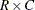tables, Fisher’s exact test is also known as the Freeman-Halton test.

JT

requests the exact Jonckheere-Terpstra test. See the sections Jonckheere-Terpstra Test and Exact Statistics for details. The JT option in the TABLES statement provides the asymptotic Jonckheere-Terpstra test.

KAPPA

requests an exact test for the simple kappa coefficient. See the sections Simple Kappa Coefficient and Exact Statistics for details. The AGREE option in the TABLES statement provides the simple kappa estimate, standard error, and confidence limits. The KAPPA option in the TEST statement provides the asymptotic test for the simple kappa coefficient.

Kappa coefficients are defined only for square tables, where the number of rows equals the number of columns. PROC FREQ does not compute kappa coefficients for tables that are not square.

KENTB

requests an exact test for Kendall’s tau-b. See the sections Kendall’s Tau-b and Exact Statistics for details. The MEASURES option in the TABLES statement provides the Kendall’s tau-b estimate and standard error. The KENTB option in the TEST statement provides an asymptotic test for Kendall’s tau-b.

LRCHI

requests an exact test for the likelihood ratio chi-square for two-way tables. See the sections Likelihood Ratio Chi-Square Test and Exact Statistics for details. For one-way tables, the LRCHI option requests an exact likelihood ratio goodness-of-fit test. See the section Likelihood Ratio Chi-Square Test for One-Way Tables for details.

The CHISQ option in the TABLES statement provides asymptotic likelihood ratio chi-square tests.

MCNEM

requests the exact McNemar’s test. See the sections McNemar’s Test and Exact Statistics for details. The AGREE option in the TABLES statement provides the asymptotic McNemar’s test.

MEASURES

requests exact tests for the Pearson and Spearman correlations. See the sections Pearson Correlation Coefficient, Spearman Rank Correlation Coefficient, and Exact Statistics for details. The PCORR and SCORR options in the TEST statement provide asymptotic tests for the Pearson and Spearman correlations, respectively.

The MEASURES option also requests exact confidence limits for the odds ratio fortables. See the sections Odds Ratio (Case-Control Studies) and Exact Confidence Limits for the Odds Ratio for details. The MEASURES and RELRISK options in the TABLES statement provide asymptotic confidence limits for the odds ratio.

MHCHI

requests an exact test for the Mantel-Haenszel chi-square. See the sections Mantel-Haenszel Chi-Square Test and Exact Statistics for details. The CHISQ option in the TABLES statement provides the asymptotic Mantel-Haenszel chi-square test.

OR

requests exact confidence limits for the odds ratio fortables. See the sections Odds Ratio (Case-Control Studies) and Exact Confidence Limits for the Odds Ratio for details. The MEASURES and RELRISK options in the TABLES statement provide asymptotic confidence limits for the odds ratio.

PCHI

requests an exact test for the Pearson chi-square for two-way tables. See the sections Pearson Chi-Square Test for Two-Way Tables and Exact Statistics for details. For one-way tables, the PCHI option requests an exact chi-square goodness-of-fit test. See the section Chi-Square Test for One-Way Tables for details. The CHISQ option in the TABLES statement provides asymptotic chi-square tests.

PCORR

requests an exact test for the Pearson correlation coefficient. See the sections Pearson Correlation Coefficient and Exact Statistics for details. The MEASURES option in the TABLES statement provides the Pearson correlation estimate and standard error. The PCORR option in the TEST statement provides an asymptotic test for the Pearson correlation.

RELRISK <(options)>

requests exact unconditional confidence limits for the relative risk fortables. PROC FREQ computes the confidence limits by inverting two separate one-sided exact tests (Santner and Snell, 1980). By default, this computation uses the unstandardized relative risk as the test statistic. If you specify the RELRISK(METHOD=SCORE) option, the computation uses the score statistic (Chan and Zhang, 1999). See the section Exact Unconditional Confidence Limits for the Relative Risk for more information.

You can set the confidence level by specifying the ALPHA= option in the TABLES statement. The default of ALPHA=0.5 produces 95% confidence limits.

The RELRISK option in the TABLES statement provides asymptotic confidence limits for the relative risk. See the section Relative Risks (Cohort Studies) for more information.

You can specify the following options in parentheses after the RELRISK statistic-option:

COLUMN=1 | 2 | BOTH

specifies thetable column for which to compute the relative risk. The default is COLUMN=1, which provides exact confidence limits for the column 1 relative risk. If you specify COLUMN=BOTH, PROC FREQ provides exact confidence limits for both column 1 and column 2 relative risks.

METHOD=SCORE

requests exact unconditional confidence limits that are based on the score statistic (Chan and Zhang, 1999). See the section Exact Unconditional Confidence Limits for the Relative Risk for more information. If you do not specify METHOD=SCORE, by default the exact confidence limit computations are based on the unstandardized relative risk.

RISKDIFF <(options)>

requests exact unconditional confidence limits for the risk difference fortables. PROC FREQ computes the confidence limits by inverting two separate one-sided exact tests (Santner and Snell, 1980). By default, this computation uses the unstandardized risk difference as the test statistic. If you specify the RISKDIFF(METHOD=SCORE) option, the computation uses the score statistic (Chan and Zhang, 1999). See the section Exact Unconditional Confidence Limits for the Risk Difference for more information.

You can set the confidence level by specifying the ALPHA= option in the TABLES statement. The default of ALPHA=0.5 produces 95% confidence limits.

The RISKDIFF option in the TABLES statement provides asymptotic confidence limits for the risk difference, including Wald, Newcombe, and Miettinen-Nurminen confidence limits. See the section Risk Difference Confidence Limits for more information.

You can specify the following options in parentheses after the RISKDIFF statistic-option:

COLUMN=1 | 2 | BOTH

specifies thetable column for which to compute the risk difference. The default is COLUMN=BOTH, which provides exact confidence limits for both column 1 and column 2 risk differences.

METHOD=SCORE

requests exact unconditional confidence limits that are based on the score statistic (Chan and Zhang, 1999). See the section Exact Unconditional Confidence Limits for the Risk Difference for more information. If you do not specify METHOD=SCORE, by default the exact confidence limit computations are based on the unstandardized risk difference.

SCORR

requests an exact test for the Spearman correlation coefficient. See the sections Spearman Rank Correlation Coefficient and Exact Statistics for details. The MEASURES option in the TABLES statement provides the Spearman correlation estimate and standard error. The SCORR option in the TEST statement provides an asymptotic test for the Spearman correlation.

SMDCR

requests an exact test for Somers’. See the sections Somers’ D and Exact Statistics for details. The MEASURES option in the TABLES statement provides Somers’estimate and the standard error. The SMDCR option in the TEST statement provides an asymptotic test for Somers’.

SMDRC

requests an exact test for Somers’. See the sections Somers’ D and Exact Statistics for details. The MEASURES option in the TABLES statement provides Somers’estimate and the standard error. The SMDRC option in the TEST statement requests an asymptotic test for Somers’.

STUTC

requests an exact test for Stuart’s tau-c. See the sections Stuart’s Tau-c and Exact Statistics for details. The MEASURES option in the TABLES statement provides Stuart’s tau-c estimate and the standard error. The STUTC option in the TEST statement provides an asymptotic test for Stuart’s tau-c.

TREND

requests the exact Cochran-Armitage test for trend. See the sections Cochran-Armitage Test for Trend and Exact Statistics for details. The TREND option in the TABLES statement provides the asymptotic Cochran-Armitage trend test. The trend test is available for tables of dimensions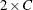or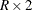.

WTKAP

requests an exact test for the weighted kappa coefficient. See the sections Weighted Kappa Coefficient and Exact Statistics for details. The AGREE option in the TABLES statement provides the weighted kappa estimate, standard error, and confidence limits. The WTKAP option in the TEST statement provides the asymptotic test for the weighted kappa coefficient.

Kappa coefficients are defined only for square tables, where the number of rows equals the number of columns. PROC FREQ does not compute kappa coefficients for tables that are not square. Fortables, the weighted kappa coefficient equals the simple kappa coefficient, and PROC FREQ does not present separate results for the weighted kappa coefficient.

Computation Options

The computation-options specify options for computing exact statistics. You can specify the following computation-options in the EXACT statement after a slash (/).

ALPHA=specifies the level of the confidence limits for Monte Carlo p-value estimates. The value ofmust be between 0 and 1, and the default is 0.01. A confidence level ofproduces% confidence limits. The default of ALPHA=.01 produces 99% confidence limits for the Monte Carlo estimates.

The ALPHA= option invokes the MC option.

MAXTIME=value

specifies the maximum clock time (in seconds) that PROC FREQ can use to compute an exact p-value. If the procedure does not complete the computation within the specified time, the computation terminates. The value of MAXTIME= must be a positive number. The MAXTIME= option is valid for Monte Carlo estimation of exact p-values as well as for direct exact p-value computation. See the section Computational Resources for more information.

MC

6 requests Monte Carlo estimation of exact p-values instead of direct exact p-value computation. Monte Carlo estimation can be useful for large problems that require a considerable amount of time and memory for exact computations but for which asymptotic approximations might not be sufficient. See the section Monte Carlo Estimation for more information.

The MC option is available for all EXACT statistic-options except the BINOMIAL option and the following options that apply only toortables: BARNARD, COMOR, EQOR, MCNEM, OR, RELRISK, and RISKDIFF. PROC FREQ computes only exact tests or confidence limits for these statistics.

The ALPHA=, N=, and SEED= options also invoke the MC option.

N=n

specifies the number of samples for Monte Carlo estimation. The value of n must be a positive integer, and the default is 10,000. Larger values of n produce more precise estimates of exact p-values. Because larger values of n generate more samples, the computation time increases.

The N= option invokes the MC option.

POINT

requests exact point probabilities for the test statistics.

The POINT option is available for all EXACT statement statistic-options except BARNARD, OR, RELRISK, and RISKDIFF. The POINT option is not available with the MC option.

SEED=number

specifies the initial seed for random number generation for Monte Carlo estimation. The value of the SEED= option must be an integer. If you do not specify the SEED= option or if the SEED= value is negative or zero, PROC FREQ uses the time of day from the computer’s clock to obtain the initial seed.

The SEED= option invokes the MC option.